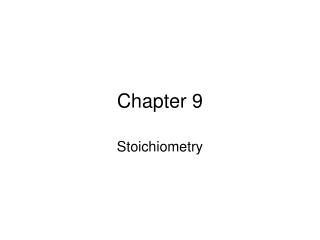DownloadDownload PresentationChapter 9

# Chapter 9

Download Presentation## Chapter 9

- - - - - - - - - - - - - - - - - - - - - - - - - - - E N D - - - - - - - - - - - - - - - - - - - - - - - - - - -
##### Presentation Transcript

1. Chapter 9 Stoichiometry

2. Stoichiometry A. The calculation of quantites in chemical reactions B. A recipe of what is happening

3. C. Interpreting Chemical Equations 1. Particles 2. Moles 3. Mass 4. Volume

4. D. N2 (g) + 3H2(g)  2 NH3 (g)

5. II. Chemical Calculations A. Mole-mole calculations 1. How many moles of ammonia are produced when 0.60 mol of nitrogen reacts with hydrogen?

6. 2. How many moles of copper are produced when 0.2 moles aluminum reacts with copper (II) sulfate? B. Mass-Mass Calculations Calculate the number of grams of NH3 produced by the reaction of 5.40 g hydrogen with an excess of nitrogen. N2(g) + 3H2 (g)  2NH3 (g)

7. Review for quiz How many grams of calcium metal will react completely with 10.0 grams of water to yield calcium hydroxide and hydrogen gas? Ca + H2O  Ca(OH)2 + H2 A reaction between methane and sulfur produces carbon disulfide. A) How many moles of CS2 are produced when 1.50 moles of S8 is used? B) How many mole H2S is produced?

8. C. Other Stoichiometric calculations 1. How many molecules of oxygen are produced when a sample of 29.2 g of water is decomposed by electrolysis?

9. 2. Assuming STP, how many liters of oxygen are needed to produce 19.8 L SO3? S(g) + O2(g)  S03(g)

10. Review for Quiz #2 How many molecules of Na2CO3 are produced from 6.7 g NaHCO3? NaHCO3 --> Na2CO3 + H2O + CO2 If carbon monoxide reacts with oxygen to produce carbon dioxide, how many liters of oxygen are required to burn 3.86 L of carbon monoxide?

11. Percent Yield A. Theoretical yield - maximum amount of product that can be produced from a given amount of reactant B. Actual yield - amount of product actually produced when the chemical reaction is carried out in an experiment

12. C. Percent yield 1. The ratio of the actual yield to the theoretical yield 2. % yield = actual yield from an exper x100 theore. Yield from calcul.

13. 3. When K2CrO4 is added to a solution containing 0.500 g AgNO3, solid silver chromate is formed. • Determine the theoretical yield of the silver chromate precipitate. • If 0.455g Ag2CrO4 is obtained, calculate the percent yield.

14. D. Limiting Reactants 1. Limiting reactants - used up first, limits the reaction 2. Excess reactants - left over reactants 3. Example: Use the reaction below, identify the limiting reactant

15. Al + O2 --> Al2O3 Find the limiting 0.25 mol .40 mol reactant. 3.6 mole Al reacts with 5.3 mole Cl2 to produce aluminum chloride.

16. c. If 14.5 moles of phosphorus reacts with 18.0 moles of oxygen to produce tetraphosphorus decoxide. d. Sodium reacts with chlorine to produce sodium chloride. 1. If 6.70 moles of Na reacts with 3.20 of Cl2 what is the limiting reactant? 2. How many moles of NaCl are produced?

17. e. How many grams of aluminum sulfide can form from the reaction of 9.00g of Al with 8.00g of S?

18. f. If 40.0 g of H3PO4 react with 60.0g of MgCO3, calculate 1. Find the limiting reactant 2. How much excess is left over 3. the grams of Mg3(PO4)2 produced 4. the grams of CO2 produced 5. the volume of CO2 produced at STP